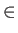Next: Lower Bounds Up: Quantum Computing Previous: Quantum Algorithms   Contents

## Error Models

Quantum computation is probabilistic in nature. We must therefore consider what (if any) error we will accept from our quantum algorithms. Different settings allow different expected running times, just as in the classical case, where different running times can be found for different types of randomized algorithms.

Three primary settings for quantum algorithms are defined by Beals et al. :

1. The Exact Setting: The quantum algorithm is required to return f (x) with certainty for every x{0, 1}N.

2. The Zero Error Setting: The quantum algorithm may be inconclusive with probability at most 1/2, but if it returns an answer, it must be correct.

3. The Bounded Error Setting: The quantum algorithm is required to return f (x) with probability at least 2/3 for every x{0, 1}N .

Clearly any algorithm in the exact setting is also correct in the bounded error setting. Algorithms in the zero error setting can be placed in the bounded error setting by performing multiple runs that return an arbitrary value whenever they would return inconclusive.'' Thus the bounded error setting is the broadest category, and as such offers the best potential speedups. All the results in this thesis refer to computations in the bounded error setting.Next: Lower Bounds Up: Quantum Computing Previous: Quantum Algorithms   Contents
Matthew Hayward Lower Query Bounds in the Quantum Oracle Model GitHub Repository